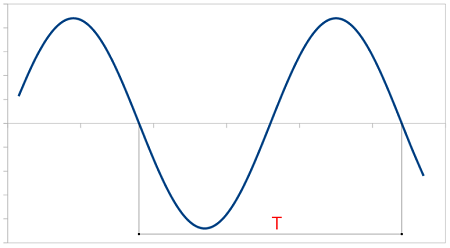Calculator of frequency from period and vice versa

Definitions

When we have an event that occurs periodically, we can define the following concepts:

Frequency.

It is the number of times that event occurs per unit time. For example, if the event consists of the movement of a pendulum, the frequency would be the number of times the pendulum passes through a given position per unit time.

Period.

It is the time that elapses between two consecutive events. That is, in the example above, it would be the time between a complete movement of the pendulum.

Calculation

To calculate the period you simply calculate the inverse of the frequency:
T = 1 /f
f = frequency
T = period
The following image shows the period of a sine wave: